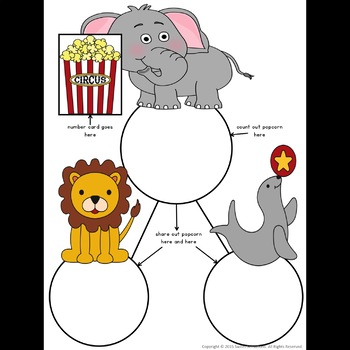# Number Bonds and Decomposing Numbers | Distance LearningK - 1st
Subjects
Standards
Resource Type
Formats Included
• PDF
Pages
7 pages

### Description

Kindergarten Operations and Algebraic Thinking to introduce composing and decomposing numbers to 10 with objects, as well as a helpful review for First Graders working with associative properties of numbers, missing addends and partitioning.

One of five activities featured in Kindergarten Composing and Decomposing Resource with Ladybug craft

and the introduction to

First Grade Number Bonds and Balancing Equations (an in-depth resource covering OA.A1-D8).

Use as a whole class, in groups or for RTI Tier II intervention.

Students need to have a firm foundation of number bonds and building and breaking numbers before they can attempt missing addends, so to begin with demonstrate using the number bonds work mats to show how to decompose and compose numbers. If possible, project this to a whiteboard and use manipulatives to show the students how to break numbers. Give each student their own work mat and popcorn manipulatives (or use counters or real popcorn!). Start by drawing one of the popcorn number cards and place that number of popcorn manipulatives onto the circle under the elephant.

Have the children share the popcorn between the lion and the seal and discuss the different ways they found to share. Then tell the students how many popcorn kernels to give the lion and have them find how many are left for the seal. Practice this frequently – it is a great way to start every math lesson and review at the end. As children become more proficient in decomposing numbers, have them play this game and record their work.

When students have shown proficiency in breaking and making numbers, they are ready to work with missing numbers and balancing equations. Students will need lots of practice.

**********************************************************************

Total Pages
7 pages
N/A
Teaching Duration
N/A
Report this Resource to TpT
Reported resources will be reviewed by our team. Report this resource to let us know if this resource violates TpT’s content guidelines.

### Standards

to see state-specific standards (only available in the US).
Decompose numbers less than or equal to 10 into pairs in more than one way, e.g., by using objects or drawings, and record each decomposition by a drawing or equation (e.g., 5 = 2 + 3 and 5 = 4 + 1).
For any number from 1 to 9, find the number that makes 10 when added to the given number, e.g., by using objects or drawings, and record the answer with a drawing or equation.
Apply properties of operations as strategies to add and subtract. If 8 + 3 = 11 is known, then 3 + 8 = 11 is also known. (Commutative property of addition.) To add 2 + 6 + 4, the second two numbers can be added to make a ten, so 2 + 6 + 4 = 2 + 10 = 12. (Associative property of addition.)
Determine the unknown whole number in an addition or subtraction equation relating three whole numbers. For example, determine the unknown number that makes the equation true in each of the equations 8 + ? = 11, 5 = ▯ - 3, 6 + 6 = ▯.